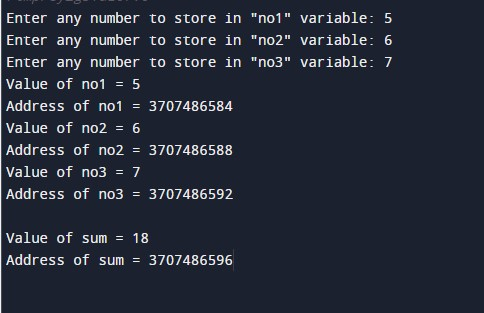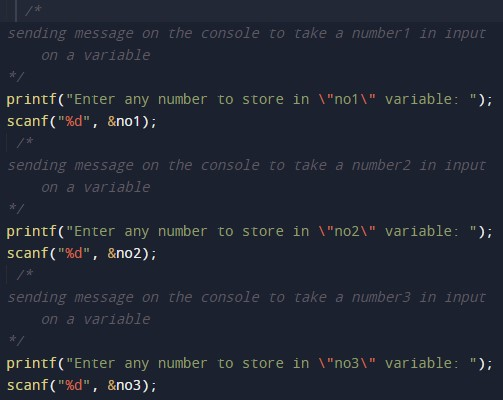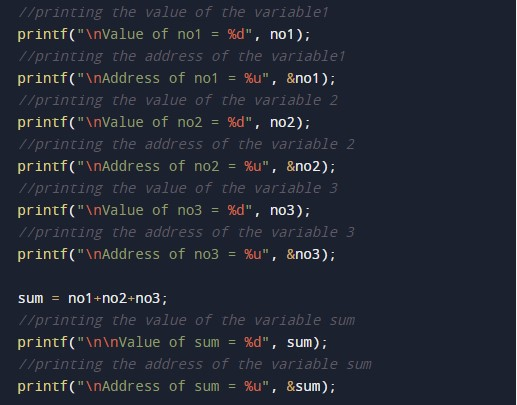C Program to print Address of Variable

In this tutorial you will learn about the C Program to print Address of Variable and its application with practical example.

C Program to print Address of Variable

In this tutorial, we will learn to create a C program that will Print the Address of the variable in C programming.

Prerequisites

Before starting with this tutorial we assume that you are best aware of the following C programming topics:

• Operators in C Programming.
• Basic Input and Output function in C Programming.
• Basic C programming.

Print the Address of the variable.

As we all know the c programming is a very powerful language. In the c programming language, we have many pre-defined functions for arithmetic operations, string functions. But today we will print the address of the variable taken input from the given number using the c programming language.

With the help of this program, we can Print the Address of the variable.

Output:-In the above program, we have first initialized the required variable.• no1 = it will hold the integer value of number input.
• no2 = it will hold the integer value of number input.
• no3 = it will hold the integer value of number input.
• sum = it will hold the integer value of number input.

Display message to Take input number from the user and scan the input number.Program code to print the data and address of the given number.In this tutorial we have learn about the C Program to print Address of Variable and its application with practical example. I hope you will like this tutorial.